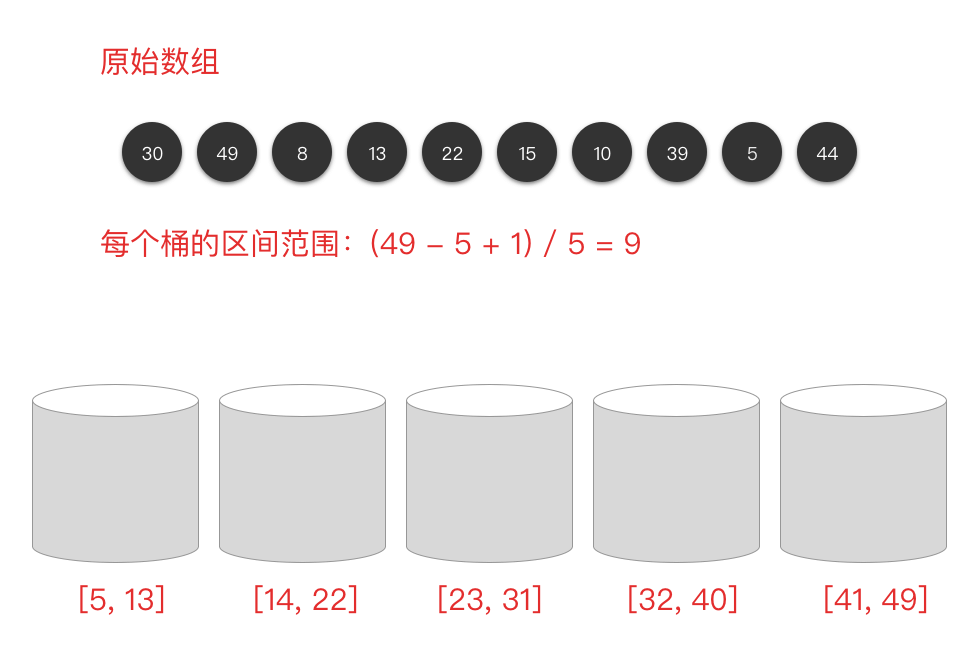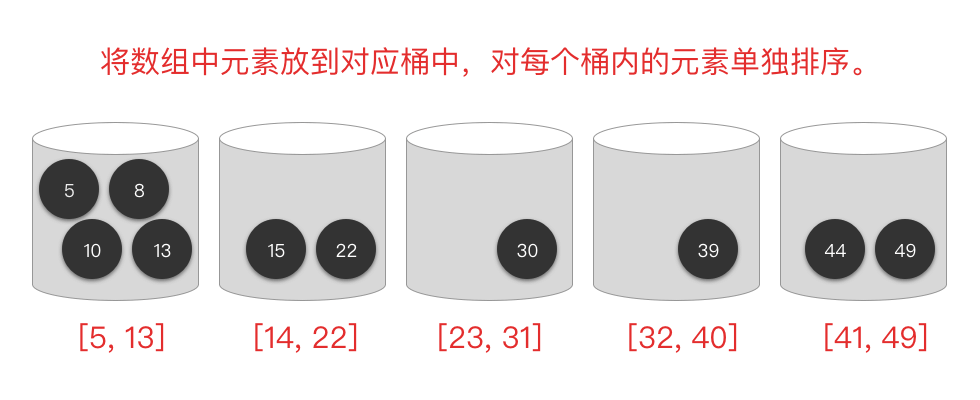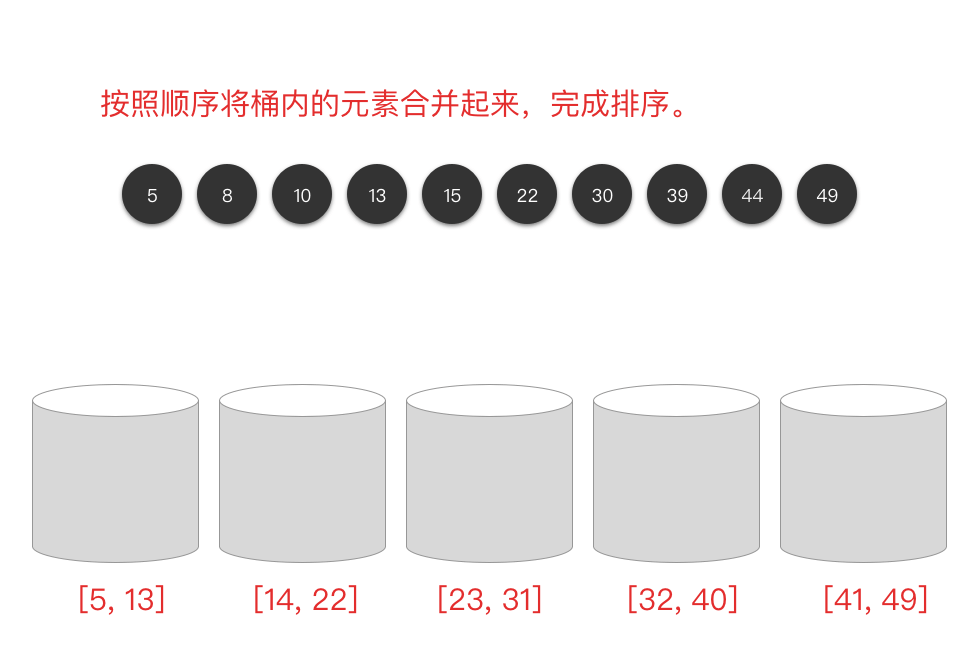09.桶排序

# 桶排序 #

## 2. 桶排序算法步骤 #

1. 根据原始数组的值域范围，将数组划分为 k 个相同大小的子区间，每个区间称为一个桶。
2. 遍历原始数组元素，将每个元素装入对应区间的桶中。
3. 对每个桶内的元素单独排序（使用插入排序、归并排序、快排排序等算法）。
4. 最后按照区间顺序将桶内的元素合并起来，完成排序。

## 3. 桶排序图解演示 #

### 3.1 划分子区间 #### 3.2 将数组元素装入桶中，并对桶内元素单独排序 #### 3.3 将桶内元素合并起来，完成排序 ### 4. 桶排序算法分析 #

• 时间复杂度：$O(n)$。当输入元素个数为 $n$，桶的个数是 $m$ 时，每个桶里的数据就是 $k = n / m$ 个。每个桶内排序的时间复杂度为 $O(k \times \log_2 k)$。$m$ 个桶就是 $m * O(k * log_2k) = m \times O((n / m) \times \log_2(n/m)) = O(n*log_2(n/m))$。当桶的个数 $m$ 接近于数据个数 $n$ 时，$log_2(n/m)$ 就是一个较小的常数，所以排序桶排序时间复杂度接近于 $O(n)$。
• 空间复杂度：$O(n + m)$。由于桶排序使用了辅助空间，所以桶排序的空间复杂度是 $O(n + m)$。
• 排序稳定性：如果桶内使用插入排序算法等稳定排序算法，则桶排序也是一种 稳定排序算法

## 5. 桶排序代码实现 #

  1 2 3 4 5 6 7 8 9 10 11 12 13 14 15 16 17 18 19 20 21 22 23 24 25 26 27 28 29 30 31 32 33 34 35 36 37 38  class Solution: def insertionSort(self, arr): # 遍历无序序列 for i in range(1, len(arr)): temp = arr[i] j = i # 从右至左遍历有序序列 while j > 0 and arr[j - 1] > temp: # 将有序序列中插入位置右侧的元素依次右移一位 arr[j] = arr[j - 1] j -= 1 # 将该元素插入到适当位置 arr[j] = temp return arr def bucketSort(self, arr, bucket_size=5): # 计算待排序序列中最大值元素 arr_max 和最小值元素 arr_min arr_min, arr_max = min(arr), max(arr) # 定义桶的个数为 (最大值元素 - 最小值元素) // 每个桶的大小 + 1 bucket_count = (arr_max - arr_min) // bucket_size + 1 # 定义桶数组 buckets buckets = [[] for _ in range(bucket_count)] # 遍历原始数组元素，将每个元素装入对应区间的桶中 for num in arr: buckets[(num - arr_min) // bucket_size].append(num) # 对每个桶内的元素单独排序，并合并到 res 数组中 res = [] for bucket in buckets: self.insertionSort(bucket) res.extend(bucket) return res def sortArray(self, nums: List[int]) -> List[int]: return self.bucketSort(nums)